# streamline

Plot streamlines from 2-D or 3-D vector data

•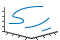## Syntax

``streamline(X,Y,Z,U,V,W,startX,startY,startZ)``
``streamline(U,V,W,startX,startY,startZ)``
``streamline(X,Y,U,V,startX,startY)``
``streamline(U,V,startX,startY)``
``streamline(verts)``
``streamline(___,options)``
``streamline(ax,___)``
``lineobj = streamline(___)``

## Description

example

````streamline(X,Y,Z,U,V,W,startX,startY,startZ)` returns plotted streamlines for 3-D vector data. The inputs `X`, `Y`, and `Z` are vector data coordinates, `U`, `V`, and `W` are vector data, and `startX`, `startY`, and `startZ` are the starting positions of the streamlines.```
````streamline(U,V,W,startX,startY,startZ)` uses the default coordinate data for `U`, `V`, and `W`. The (x,y,z) location for each element in `U`, `V`, and `W` is based on the column, row, and page index, respectively.```

example

````streamline(X,Y,U,V,startX,startY)` returns plotted streamlines for 2-D vector data. The inputs `X` and `Y` are vector data coordinates, `U` and `V` are vector data, and `startX` and `startY` are the starting positions of the streamlines.```
````streamline(U,V,startX,startY)` uses the default coordinate data for  `U` and `V`. The (x,y) location for each element in `U` and `V` is based on the column and row index, respectively. ```

example

````streamline(verts)` plots streamlines from vertices, specified as a cell array of vertex arrays (as returned by `stream2`, `stream3`, or `streamslice`).```
````streamline(___,options)` plots streamlines using the specified options, defined as a one- or two-element vector with the form `step` or `[step maxvert]`, where `step` is the step size in data units for interpolating the vector data and `maxvert` is the maximum number of vertices in a streamline. Use this argument with any of the input argument combinations from the previous syntaxes. ```
````streamline(ax,___)` plots streamlines into the specified axes, instead of into the current axes object (`gca`). ```

example

````lineobj = streamline(___)` returns a vector of one or more `Line` objects. Use `lineobj` to modify properties of the streamlines after creating them. For a list of properties, see Line Properties.```

## Examples

collapse all

Load the `wind` data set which contains measurements of air current over regions of North America.

• 3-D arrays `x`, `y`, and `z` represent the locations of air current measurements.

• 3-D arrays `u`, `v`, and `w` represent the velocity of the air current in 3-D vector fields.

Define the starting position of 16 hypothetical particles. In this case, the particles all start at `x` = 80 and have starting `y` positions ranging from 20 to 50 and starting `z` positions ranging from 0 to 15.

```load wind [startX,startY,startZ] = meshgrid(80,20:10:50,0:5:15);```

Compute the 3-D streamline vertex data for a hypothetical particle placed into the air current at the collection of starting positions in `startX`, `startY`, and `startZ`.

`verts = stream3(x,y,z,u,v,w,startX,startY,startZ);`

Visualize the 3-D volume of vector fields with `streamline`. Return the line objects in the variable `lineobj`, so you can change their properties later.

```lineobj = streamline(verts); view(3)```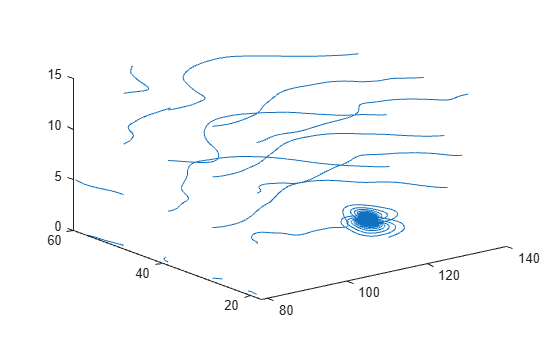To change aspects of a particular line, set properties on one of the returned line objects. For example, change the color of the tenth line to green and change its thickness to `3`.

```lineobj(10).Color = "g"; lineobj(10).LineWidth = 3;```Load the `wind` data set, which contains measurements of air current over regions of North America.

• 3-D arrays `x` and `y` represent the locations of air current measurements.

• 3-D arrays `u` and `v` represent the velocity of the air current in 3-D vector fields.

Use the fifth page of the arrays. Define the starting position of four hypothetical particles. In this case, the four starting locations are (80, 20), (80, 30), (80, 40), and (80, 50).

```load wind x5 = x(:,:,5); y5 = y(:,:,5); u5 = u(:,:,5); v5 = v(:,:,5); [startX,startY] = meshgrid(80,20:10:50);```

Compute the 2-D streamline vertex data for a hypothetical particle placed into the air current with `stream2`.

`verts = stream2(x5,y5,u5,v5,startX,startY);`

Visualize the 2-D matrix of vector fields by calling `streamline`. Return the line objects in the variable `lineobj`, so you can change their properties later.

`lineobj = streamline(verts);`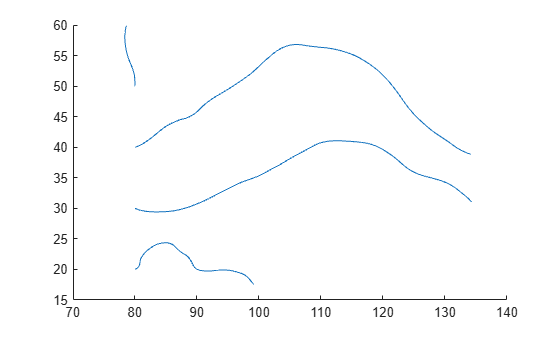To change aspects of a particular line, set properties on one of the returned line objects. For example, change the color of the second line to magenta and change its style to dashed.

```lineobj(2).Color = "m"; lineobj(2).LineStyle = "--";```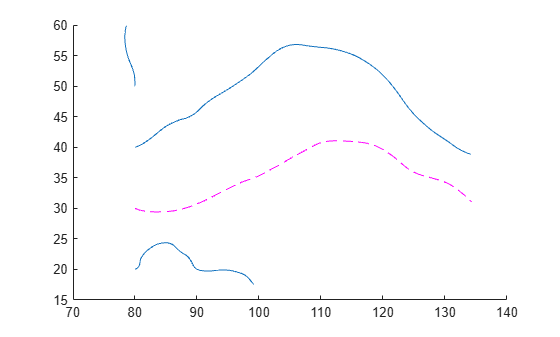Load the `wind` dataset and compute the vertices of streamlines that start at evenly spaced points on the plane `x = 80`. Then, plot the streamlines from the vertex data.

```load wind [startX,startY,startZ] = meshgrid(80,20:10:50,0:5:15); verts = stream3(x,y,z,u,v,w,startX,startY,startZ); streamline(verts) axis tight view(3);```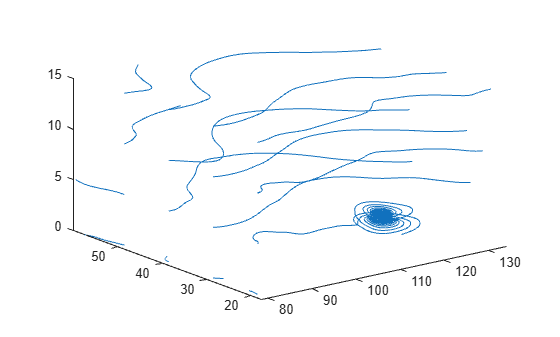## Input Arguments

collapse all

x-axis coordinates of vector data, specified as a 2-D or 3-D array that can be combined with `Y` (and optionally `Z`) to form a grid of coordinates. You can use the `meshgrid` function to create the arrays.

`X` must be the same size as `Y`, `Z`, `U`, `V`, and `W`.

y-axis coordinates of vector data, specified as a 2-D or 3-D array that can be combined with `X` (and optionally `Z`) to form a grid of coordinates. You can use the `meshgrid` function to create the arrays.

`Y` must be the same size as `X`, `Z`, `U`, `V`, and `W`.

z-axis coordinates of vector data, specified as a 3-D array that can be combined with `X` and `Y` to form a grid of coordinates. You can use the `meshgrid` function to create the arrays.

`Z` must be the same size as `X`, `Y`, `U`, `V`, and `W`.

x-components of vector data, specified as a 2-D or 3-D array. `U` must be the same size as `X`, `Y`, `Z`, `V`, and `W`.

y-components of vector data, specified as a 2-D or 3-D array. `V` must be the same size as `X`, `Y`, `Z`, `U`, and `W`.

z-components of vector data, specified as a 3-D array. `W` must be the same size as `X`, `Y`, `Z`, `U`, and `V`.

x-axis streamline starting positions, specified as a vector or matrix. `startX` must be a scalar or be the same size as `startY` and `startZ`.

y-axis streamline starting positions, specified as a vector or matrix. `startY` must be a scalar or be the same size as `startX` and `startZ`.

z-axis streamline starting positions, specified as a vector or matrix. `startZ` must be a scalar or be the same size as `startX` and `startY`.

Streamline vertices, specified as a cell array (as returned by `stream2`, `stream3`, or `streamslice`). Each element of the cell array is a matrix of vertices for one line.

Streamline options, specified as a one- or two-element vector with one of the following forms:

• `step`

• `[step,maxvert]`

`step` is the step size used to adjust the streamline resolution and determine the vertex locations for which streamline velocity is interpolated. `maxvert` is the maximum number of vertices calculated for a streamline before computation is complete.

The default step-size is `0.1`, and the default maximum number of vertices is `10,000`.

Target axes, specified as an `Axes` object. If you do not specify the axes, then the `streamline` function uses the current axes.

## Version History

Introduced before R2006a

expand all